## A denoising autoencoder for CIFAR dataset(s)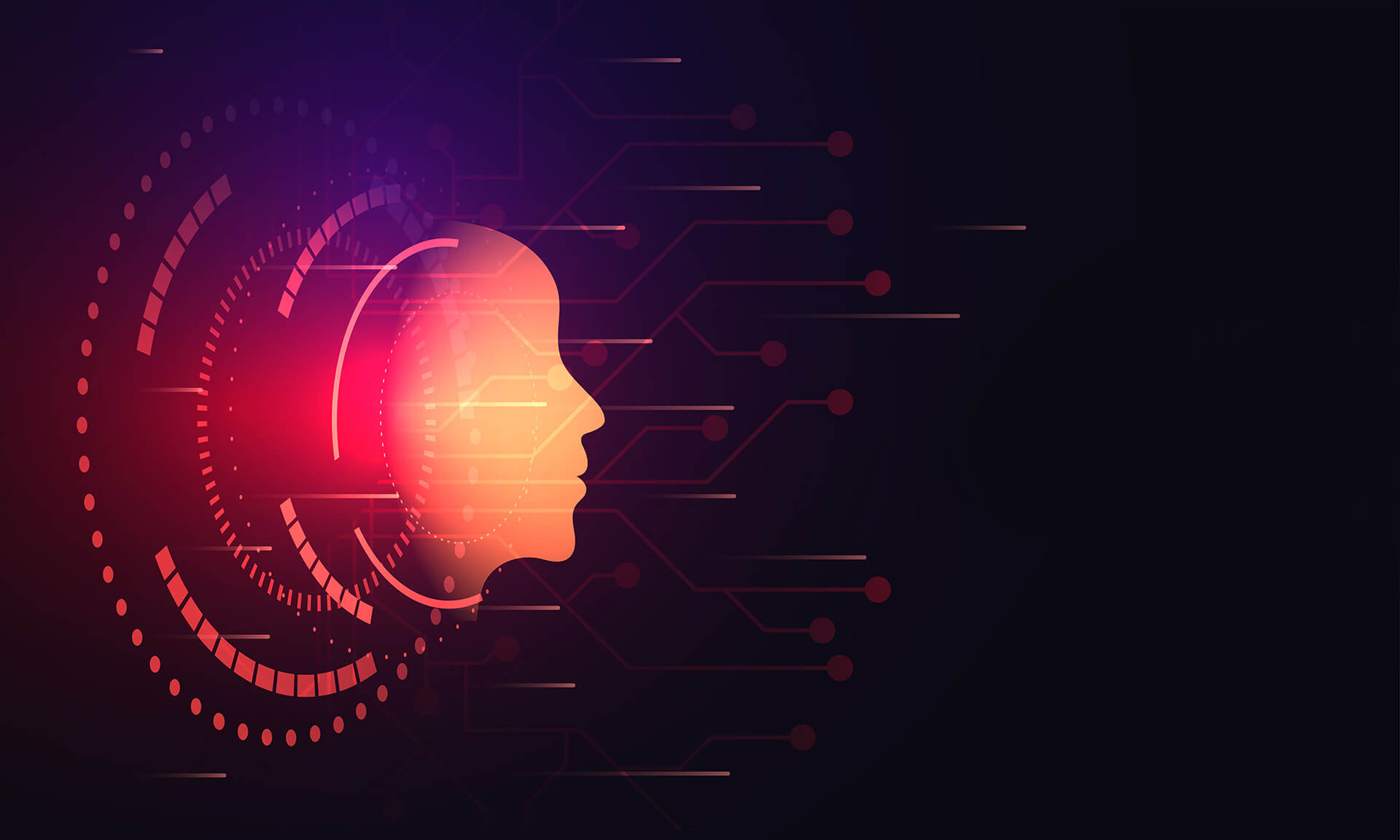Every once in a while we come across an image on our shelf that we like, but sometimes that image is stained by coffee, markers or simply time. What can we do if we want the original image back, without those smears?

What can help us is called exactly like the title suggests: a denoising autoencoder (DAE later in this article). But what exactly is this denoising autoencoder?

Let’s start with autoencoder. An autoencoder is a neural network that consists of 2 parts: an encoder and a decoder. An exemplary image of such a network can be seen below.

The job of encoders is to reduce the dimensionality of our data (just like PCA). That data is then recreated by the decoder. The most basic autoencoder is trained by comparing original data put into the encoder’s input and comparing it with the decoder’s output. For DAEs we put noisy data into the encoder’s input and compare the decoder’s output with clean data. For some tasks we train the whole autoencoder and later only use the encoder part to get rid of unnecessary variables. But here we will use both parts of the network.

In this short article we’ve decided to try to train the DAE on a CIFAR100 dataset and check how well it denoises images from this CIFAR10 dataset. The deep learning library of our choice for this task was Keras.

First some imports

``````import numpy as np
import matplotlib.pyplot as plt
from tensorflow.keras.layers import Conv2D, Input, Dense, Reshape, Conv2DTranspose,\
Activation, BatchNormalization, ReLU, Concatenate
from tensorflow.keras.models import Model
from tensorflow.keras.callbacks import ModelCheckpoint
from tensorflow.keras.datasets import cifar100, cifar10``````

Then we load the CIFAR100 dataset, more about it and CIFAR10 can be found here. Probably the most important point is that none of the images of CIFAR100 can be found in the CIFAR10 dataset and vice versa.

We don’t load labels, because we don’t care about them at all.

``(train_data_clean, _), (test_data_clean, _) = cifar100.load_data(label_mode='fine')``

Next step: convert data to floats 0-1.

``````train_data_clean = train_data_clean.astype('float32') / 255.
test_data_clean = test_data_clean.astype('float32') / 255.``````

Now we need to produce noisy data. To do this we add gaussian noise with mean=0 and std=0.1 and then clip values back to 0-1. Mean=0 noise makes some parts of the image darker and some lighter after addition.

``````def add_noise_and_clip_data(data):
noise = np.random.normal(loc=0.0, scale=0.1, size=data.shape)
data = data + noise
data = np.clip(data, 0., 1.)
return data
​

Quick check if everything is alright.

``````idx = 4
plt.subplot(1,2,1)
plt.imshow(train_data_clean[idx])
plt.title('Original image')
plt.subplot(1,2,2)
plt.imshow(train_data_noisy[idx])
plt.title('Image with noise')
plt.show()``````

## Part 2: Defining the architecture

Now we define the building blocks of our DAE: a convolutional block and a deconvolutional block.

Convolutional blocks consist of 3 operations: 2D convolution, batch normalization and ReLu activation. We use strides=2 to downsample data going through the network.

Deconvolutional blocks also consist of 3 operations: 2D transposed convolution, batch normalization and also ReLu activation. Here strides=2 is used to upsample the data.

``````def conv_block(x, filters, kernel_size, strides=2):
x = Conv2D(filters=filters,
kernel_size=kernel_size,
strides=strides,
x = BatchNormalization()(x)
x = ReLU()(x)
return x
​
def deconv_block(x, filters, kernel_size):
x = Conv2DTranspose(filters=filters,
kernel_size=kernel_size,
strides=2,
x = BatchNormalization()(x)
x = ReLU()(x)
return x``````

Our model architecture is inspired by U-Net:

• 4 convolutional blocks with downsampling
• 1 convolutional block without downsampling
• 4 deconvolutional blocks with upsampling, interleaving concatenations
• 1 final deconvolution that recreates image size (32, 32, 3)
• 1 activation layer with sigmoid that scales values to 0-1.

Of course we encourage you to try your own archs, something like DenseNet’s skip connections may perform well in our task.

``````def denoising_autoencoder():
dae_inputs = Input(shape=(32, 32, 3), name='dae_input')
conv_block1 = conv_block(den_inputs, 32, 3)
conv_block2 = conv_block(conv_block1, 64, 3)
conv_block3 = conv_block(conv_block2, 128, 3)
conv_block4 = conv_block(conv_block3, 256, 3)
conv_block5 = conv_block(conv_block4, 256, 3, 1)
​
deconv_block1 = deconv_block(conv_block5, 256, 3)
merge1 = Concatenate()([deconv_block1, conv_block3])
deconv_block2 = deconv_block(merge1, 128, 3)
merge2 = Concatenate()([deconv_block2, conv_block2])
deconv_block3 = deconv_block(merge2, 64, 3)
merge3 = Concatenate()([deconv_block3, conv_block1])
deconv_block4 = deconv_block(merge3, 32, 3)
​
final_deconv = Conv2DTranspose(filters=3,
kernel_size=3,
​
dae_outputs = Activation('sigmoid', name='dae_output')(final_deconv)

return Model(dae_inputs, dae_outputs, name='dae')``````

## Part 3: Training the model

Now we compile the model using mean squared error as our loss and Adam as the optimizer. ModelCheckpoint callback saves currently the best model during training.

And finally we train the model for 40 epochs. Using batch_size=128 best val_loss is around 0.199.Note: If you don’t want to train the model yourself we provide our best model from this training.

``````dae = denoising_autoencoder()
​
checkpoint = ModelCheckpoint('best_model.h5', verbose=1, save_best_only=True, save_weights_only=True)
​
dae.fit(train_data_noisy,
train_data_clean,
validation_data=(test_data_noisy, test_data_clean),
epochs=40,
batch_size=128,
callbacks=[checkpoint])``````

## Part 4: Interpreting the results

Now we load our best model weights and try to predict (denoise) our CIFAR100 test data to visualize how well our DAE performs. After all, a loss number doesn’t mean much if that’s all we see.Note: if you didn’t train the model yourself change ‘best_model.h5’ to ‘pretrained_model/best_model.h5’.

``````dae.load_weights('best_model.h5')
test_data_denoised = dae.predict(test_data_noisy)
idx = 4
plt.subplot(1,3,1)
plt.imshow(test_data_clean[idx])
plt.title('original')
plt.subplot(1,3,2)
plt.imshow(test_data_noisy[idx])
plt.title('noisy')
plt.subplot(1,3,3)
plt.imshow(test_data_denoised[idx])
plt.title('denoised')
plt.show()``````

Now we will calculate the mean squared error of the whole CIFAR100 test set. First we will calculate the mse between our clean data and the data with added noise. Next we check how well our DAE denoised the data.

``````def mse(data_1, data_2):
return np.square(np.subtract(data_1, data_2)).mean()
​
noisy_clean_mse = mse(test_data_clean, test_data_noisy)
denoised_clean_mse = mse(test_data_denoised, test_data_clean)

noisy_clean_mse, denoised_clean_mse``````

Output:

``(0.0091120318424328, 0.0015556107)``

As you can see, our DAE decreased the noise around 6x (by ~83%).

## Part 5: Testing our DAE on CIFAR10

As previously we load the data, only images.

``(cifar10_train, _), (cifar10_test, _) = cifar10.load_data()``

Convert images to floats and add noise.

``````cifar10_train = cifar10_train.astype('float32') / 255.
cifar10_test = cifar10_test.astype('float32') / 255.

And now we can denoise these images.

``cifar10_test_denoised = dae.predict(cifar10_test_noisy)``

And just like before, we plot our results.

``````idx = 6
plt.subplot(1,3,1)
plt.imshow(cifar10_test[idx])
plt.title('original')
plt.subplot(1,3,2)
plt.imshow(cifar10_test_noisy[idx])
plt.title('noisy')
plt.subplot(1,3,3)
plt.imshow(cifar10_test_denoised[idx])
plt.title('denoised')
plt.show()``````
``````clean_noisy = mse(cifar10_test, cifar10_test_noisy)
clean_denoised = mse(cifar10_test, cifar10_test_denoised)

clean_noisy, clean_denoised``````

Output:

``(0.009305541238191785, 0.001531884)``

Seeing the number, we can tell that our DAE worked pretty much as well on CIFAR10 as on CIFAR100. Images in both datasets aren’t that different after all and the image resolution is low.

## Conclusion

The DAE fulfilled its task. After training on CIFAR100, it denoised CIFAR10 images without problems and without a substantial drop in quality.Bartosz Fijalkowski

Ruby on Rails, AWS Dev ops

## Interested in collaborating?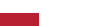Codahead implements a project co-financed from European Funds "Promotion of services provided by Codahead in selected foreign markets." The aim of the project is to develop the company's export activities in foreign markets.Top B2B Companies
Poland 2019## Get an Estimate

####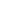Rydlówka 20, 30-363 Kraków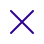What would you like to do?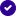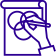Build something new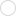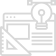Improve existing project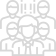Extend my team

What is the scope of your project?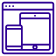Software Development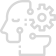AI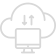IoT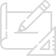Product Design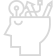Others